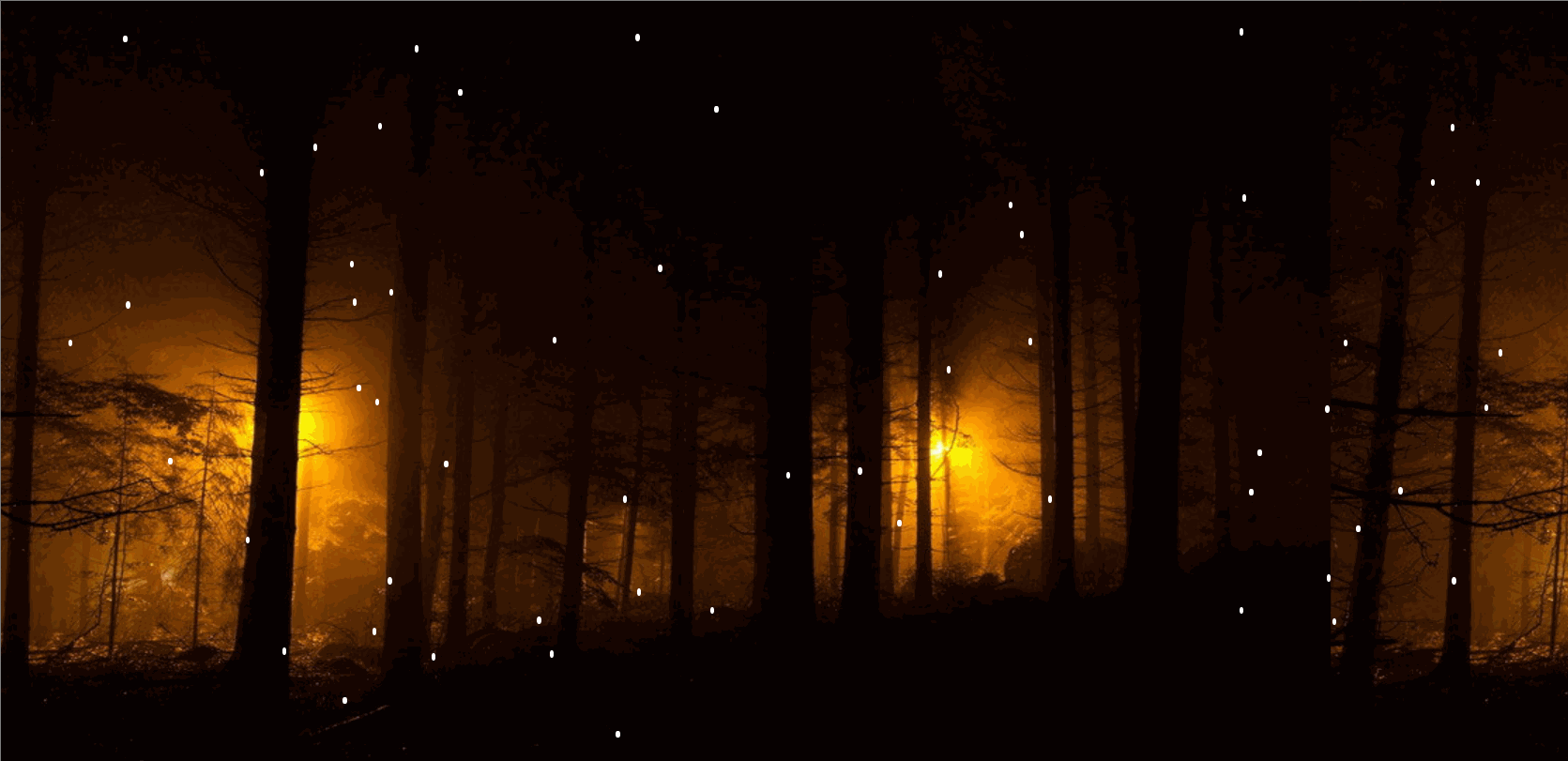# 原生JS实现萤火虫效果

更新时间：2020年03月07日 10:30:16   作者：huangfuyk.CSS部分（此处用元素模拟萤火虫，背景可自行设置）：

```<style>
.box{width: 4px;height: 5px;background: wheat;position: absolute;border-radius: 50%;}
body{background: url(../img/bg.jpg) ;}
</style>```

JS部分：

```<script>
class Glowworm{
constructor(){
// 获取屏幕的可视区域的宽高，用作将来的随机范围
this.clientW = document.documentElement.clientWidth;
this.clientH = document.documentElement.clientHeight;
// 假设萤火虫的宽高
this.w = 20;
this.h = 20;
}
createEle(){
var div = document.createElement("div");
div.className = "box";
document.body.appendChild(div);
// 在创建元素之前一定得先生成随机坐标
div.style.left = this.x + "px";
div.style.top = this.y + "px";
// 元素创建好之后，立即运动
this.move(div);
}
randomPos(){
// 随机生成坐标
this.x = random(0,this.clientW - this.w);
this.y = random(0,this.clientH - this.h);
}
move(ele){
// 开始运动之前，还得随机生成目标
this.randomPos();
// 开始运动
move(ele,{
left:this.x,
top:this.y
},()=>{
// 一个动画结束后，重复开启当前动画，即可
this.move(ele);
})
}
}

for(var i=0;i<60;i++){
// 先得到实例
var g = new Glowworm();
// 生成随机坐标
g.randomPos();
// 再创建元素
g.createEle();
}

function random(a,b){
return Math.round(Math.random()*(a-b)+b);
}
</script>```

```function move(ele,obj,cb){
clearInterval(ele.t);
ele.t = setInterval(() => {
var i = true;
for(var attr in obj){
if(attr == "opacity"){
var iNow = getStyle(ele,attr) * 100;
}else{
var iNow = parseInt(getStyle(ele,attr));
}
let speed = (obj[attr] - iNow)/10;
speed = speed < 0 ? Math.floor(speed) : Math.ceil(speed);
// 只要有一个属性没到目标：绝对不能清除计时器
if(iNow !== obj[attr]){
i = false;
}
if(attr == "opacity"){
ele.style.opacity = (iNow + speed)/100;
}else{
ele.style[attr] = iNow + speed + "px";
}
}
if(i){
clearInterval(ele.t);
if(cb){
cb();
}
// cb && cb();
}
}, 30);
}

function getStyle(ele,attr){
if(ele.currentStyle){
return ele.currentStyle[attr];
}else{
return getComputedStyle(ele,false)[attr];
}
}```